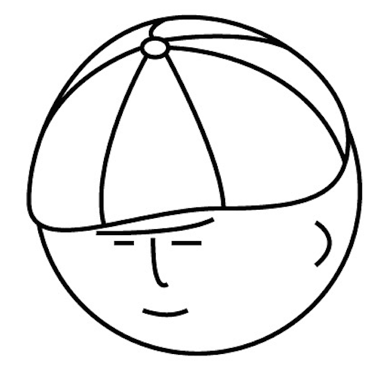マツシタのお勉強メモ

マツシタのお勉強

welcome to my engineering blog
Hacker RankMaximum Subarray

Problem Find the contiguous subarray within an array (containing at least one number) which has the largest sum. For example, given the array [-2,1,-3,4,-1,2,1,-5,4], the contiguous subarray [4,-1,2,1] has the largest sum = 6. leetcode.com…

Robot is moving in grid

Problem Imagine a robot sitting on the upper left corner of an X by Y grid. The robot can only move in two directions: right and down. How many possible paths are there for the robot to go from (0, 0) to (X, Y)? How to Solve By using Dynam…

361. Bomb Enemy : Past Google Coding Interview

Problem https://leetcode.com/problems/bomb-enemy/ How to Solve This problem can be solved by using Dynamic Programming. The point of this problem is that one spot in the grid can divide into four direction (up, down, left, right). We can c…